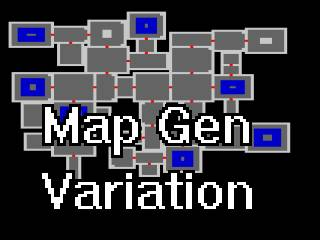-=+=- -=+=- -=+=- -=+=- -=+=- -=+=- -=+=- -=+=- -=+=- -=+=- -=+=- -=+=- -=+=- -=+=- -=+=- -=+=- -=+=- -=+=- -=+=- -=+=- -=+=- -=+=- -=+=- -=+=- -=+=- -=+=- -=+=- -=+=- -=+=- -=+=- (c) WidthPadding Industries 1987 0|303|0 -=+=- -=+=- -=+=- -=+=- -=+=- -=+=- -=+=- -=+=- -=+=- -=+=- -=+=- -=+=- -=+=- -=+=- -=+=- -=+=- -=+=- -=+=- -=+=- -=+=- -=+=- -=+=- -=+=- -=+=- -=+=- -=+=- -=+=- -=+=- -=+=- -=+=-
SoCoder -> Showcase Home -> Graphic Demos

PakzCreated : 16 June 2016
Edited : 19 June 2016
System : Cross Platform
Language : Monkey

### Map Generator variation

Rooms and doors and things in rooms

ScreenshotsI am stil learning how to be able to make levels and make them interesting. Here is one of the last things I did.

The code creates rooms with doors and puts things in the rooms sometimes(water or walls)

The link is to a html5 page where a level is generated every second. There is also a link to the code on github.

## Latest Update

I turned the code into a fps in b3d :

https://cromdesi.home.xs4all.nl/games/fpsrandommap/fpsrandommap.7z

Friday, 17 June 2016, 08:27
HoboBenOooh that's really neat!What technique did you use? Is there a name for it?
Saturday, 18 June 2016, 00:08
PakzI learned this over time from examples from the internet (bb.com and rogue basin ect.) Not sure if there are names for the methods that I used. Did not see any method names in the examples.

I used the random command to place the rooms and doors, size. The things in the rooms are based on if there is enough size to place anything in them.

One tutorial I found once had a technique that probably got a name(I cant find it anymore) is where you have parts(segments?) of the map and place them next to each other if they can be put next to it. Ending up with a random map. This was something that helped me understand map generation more. No idea where I read it though.